## //----------------------------// ArtMatic 2 in 5 out components //----------------------------//

Introduction
Disk Moebius Trap
Polar Space
Rotate N
Mirror
Mirror N
Outer Mirror N
Tiles
Hexa Mirror Tiles
Mirror Tiles
Spiral Polar Tile
Appolonian Tile
Disk Inversion
Outer Disk Mirror
Disks & Plane Inversion
Soddy Inversions
O Soddy Inversions
Inversion Ring #
Disk Modulo #
Polar Spiral Band
Escher Disk Tile
Random Twirls
Multi Perlin displace
Disk Fractal Lab
Fractal Polar Tile
Kleinian Group Fractal #
Fractal Ring
Moebius Discs #
Moebius Balls
Disk Inversion Spiral #
Moebius Inversions
Random disk packing
25 Compiled tree

## Introduction

25 components are unique in that they act in many ways like a composite function that combines two functions with independent outputs (a space transform and a color texture generator) in one tile. When the input is a 2D space, the left two outputs are a space transform and the right three outputs are an RGB output texture. In many cases, you will use only the left two outputs or the rightmost outputs. When a 25 component is the bottom component of a tree its three right outputs are used for RGB output and the left two outputs are ignored.

##25 Disk Moebius Trap

parameters :
A : K factor (-0.99 : 0.99)
B : Offset x (-8. : 8.)
C : Offset y (-8. : 8.)
D : Rotation (-180. : 180.)

discussion :
Implements a parabolic Moebius transform that keeps the unit disk invariant. The "K factor" basically changes the amount of curvature. The tile Algorithm specifies if infinity will be mapped inside or outside the Unit disk.
The example Libraries/Maths/Complex maths/Unit Moebius trap K factor shows how the unit square corners stays on the unit disk while changing the K factor.
The example Libraries/Maths/Complex maths/Unit Moebius trap iter shows how iterative transform of a (0,-2) disk maps the disks tangentially to the Unit disk;

algorithms :
• inf Inside Unit disc:
infinity will be mapped inside the Unit disk.
• inf Outside Unit disc :
infinity will be mapped outside the Unit disk.

##25 Polar Space

parameters :
A : Radial steps # (2. : 32.)
B : Radial phase (-8. : 8.)
C : Phase (-8. : 8.)

discussion :
Space transform. Polar transform of the input space. Polar transforms turn straight lines into curves and circles as demonstrated by the remapping of the Grid. RGB texture. A circular mapping of the current gradient uninfluenced by the component parameters:

• A: Radial Steps. The number of axes of symmetry.
• B: Radial Phase. Rotation of the output space.
• C: Phase. This parameter shifts the input space to or away from the center.

##25 Rotate N

parameters :
A : Rotation steps # (2 : 31)
B : Phase (-16. : 16.)
C : Scale (1. : 8.)

discussion :
Space transform - Kaleidoscopic space transform that takes a wedge of the incoming space and repeats it by rotating it around the center. The number of wedges created is determined by parameter A.

RGB texture - Wedges colored by the active gradient. Rotation Steps. The number of axes of symmetry. This influences both the color texture and the space transform.

##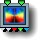25 Mirror

parameters :
A : Rotation (-180. : 180.)
B : Offset x (-32. : 32.)
C : Offset y (-32. : 32.)

discussion :
Space transform - Mirrors the incoming space about an axis whose rotation is controlled by parameter A. The transform is equivalent to
2D Mirror tile.

RGB texture - Mirrored color wheel drawn from the current gradient and mirrored with the same rotation as the space transform. A line is drawn with the first auxiliary color at the center of the mirror.

##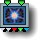25 Mirror N

parameters :
A : Symmetries # (2 : 36)
B : Offset x (-32. : 32.)
C : Offset y (-32. : 32.)

discussion :
Space transform - Mirror the incoming space with N number of axes of symmetry for kaleidoscopic effects. The transform is equivalent to
N-Fold Mirrors # when mirroring inward.

RGB texture - Mirrored color wheel drawn from the current gradient and mirrored with the same rotation as the space transform. A line is drawn with the first auxiliary color for each axis.

##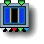25 Outer Mirror N

parameters :
A : Rotation (-180. : 180.)
B : Offset x (0. : 16.)
C : Mirrors number (1 : 12)

discussion :
Space transform - This component mirrors the incoming space outwards with the number of symmetries determined by the Mirrors Number parameter. Parameters A and B allow a rotation and offset to be applied before the mirroring is performed. The result is like a kaleidoscope on steroids. Be sure to explore how rotation, offset and the mirrors number interact.

RGB texture - Wedges of color taken from the current gradient. The number of wedges is determined by parameter A.

##25 Tiles

parameters :
A : Scale (0. : 16.)
B : Frequency (0. : 16.)
C : Scale Y (0. : 2.)

discussion :
Space Transform - Tile the incoming space into a matrix of rectangular tiles.

RGB texture - An arrangement of identically-sized rectangular tiles filled with colors from the current gradient and highlighted by the first auxiliary color.

Scale Y - Adjusts the tile height without affecting the width. Use this parameter to create non-square tiling.

##25 Hexa Mirror Tiles

parameters :
A : Scale (0. : 16.)
B : Frequency (0. : 16.)
C : Phase (0. : 2.)

discussion :
Space transform - Tile space into kaleidoscope-like arrangements of hexagonally-symmetric hexagonal tiles.

RGB texture - Colored hexagonal tiles filled with colors taken from the current gradient and outlined with the first auxiliary color.

##25 Mirror Tiles

parameters :
A : Scale (0. : 16.)
B : Frequency (0. : 16.)
C : Scale Y (0. : 2.)

discussion :
Space transform - Tile the space into identical mirrored tiles. This performs the same operation as the 22 Orthogonal Mirror Tiles component.

RGB texture - An arrangement of identically-sized rectangular tiles that are filled with colors from the current gradient and highlighted by the first auxiliary color.

Scale Y - Adjusts the tile height without affecting the width. Use this parameter to create non-square tiling.

##25 Spiral Polar Tile

parameters :
A : Size (0. : 1.)
B : Tile # (1. : 32.)
C : Phase (-32. : 32.)

discussion :
Space transform - Encapsulates the incoming space in tiles arranged in an infinite inward logarithmic spiral.

RGB texture - Circles colored by the active gradient that co-incide with the space transform's tiles.##25 Appolonian Tile

parameters :
A : Radius (0. : 4.)
B : Offset x (-4. : 4.)
C : Offset y (-4. : 4.)

discussion :
Space transform - Remap the incoming space into an arrangement of circular tiles that tries to fill space with non-overlapping circles of smaller and smaller size using the

RGB texture - Circles colored with the colors of the current gradient and first auxiliary color are presented in a pattern that co-incides with the space transform's tiles.

##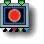25 Disk Inversion

parameters :
A : Inversion Radius (0. : 8.)
B : Offset x (-8. : 8.)
C : Offset y (-8. : 8.)

discussion :
Space transform - Inward mirroring of the space using a disk-based inversion. The transform is similar to the
22 Disk Mirror when mirror concavity is 1.

RGB texture - A red disk outlined with the first auxiliary color in disk frame mode.

Options:

• "Plain disk"
• "Disk frame" Outlines the main disk and the line y=O
• "Disk & Inversions frame " Outlines the inversions as well as the main disk and the line at y=O

##25 Outer Disk Mirror

parameters :
A : Inversion Radius (0. : 8.)
B : Offset x (-8. : 8.)
C : Offset y (-8. : 8.)

discussion :
Space transform. Disk-based mirror that reflects the inner-portion of the space outwards. The transform is similar to the
22 Disk Mirror when mirror concavity is 0.

RGB texture. A green disk outlined with the first auxiliary color.

Options:

• "Plain disk"
• "Disk frame" Outlines the main disk and the line y=O
• "Disk & Inversions frame " Outlines the inversions as well as the main disk and the line at y=O
See Disk Inversions

##25 Disks & Plane Inversion

parameters :
A : Inversion Radius (0. : 8.)
B : Offset x (-8. : 8.)
C : Offset y (-8. : 8.)

discussion :
Does a mirror in y axis and a 4 disks inversion and shade the plane y<0 in blue and the unit disk in red. Disks & Plane Inversion has the following shading option :

• "Plain disk"
• "Disk frame" Outlines the main disk and the line y=O
• "Disk & Inversions frame " Outlines the inversions as well as the main disk and the line at y=O

##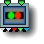25 Soddy Inversions

parameters :
A : Radius (0. : 8.)
B : Offset x (-4. : 4.)
C : Offset y (-4. : 4.)

discussion :
Space transform. A two-dimensional implementation of Soddy's Hexlet to mirror the incoming space. Two tangent mirroring circles are drawn and the space is mirrored inwardly.

Example: On the Y axis two infinite planes lies tangent to the circles upward and downward. When incoming space is inverted with 22 complex inversion and x,y offset is non zero you will obtain mutually tangent circles in a disk (Soddy cirles).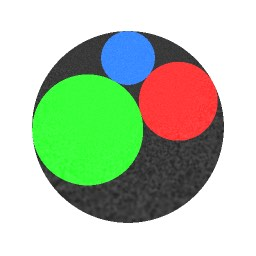Example: the space is mirrored inwardly into each new circle. If the transform is applied recursively it will create a set of smaller discs outlining an Apollonian disc packing as shown below :##25 O Soddy Inversions

parameters :
A : Radius (0. : 8.)
B : Offset x (-4. : 4.)
C : Offset y (-4. : 4.)

discussion :
Space transform - Tangents Mirroring circles of "Radius" A are placed at infinity on the vertical axis. When incoming space is inverted with
22 complex inversion and x offset is non zero you will obtain mutually tangent circles in a disk. If the transform is applied recursively it will create an Apollonian disk packing. See Apollonian Gasket.RGB texture - Circles The first and second auxiliary colors are used for the left and right regions while other circles are using primaries.

Options:

• "Plain disk"
• "Disk frame"
• "Disk & Inversions frame"

##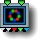25 Inversion Ring #

parameters :
A : Radius (0.06 : 12.)
B : Tile # (2 : 16)
C : Offset y (-8. : 8.)

discussion :
Space transform - N Tangents Mirroring circles of "Radius" A are arranged in a ring. "Tiles" sets N, the number of inverting disks.

RGB texture - circles of pre-determined color are drawn to correspond with the tiles. Various Disk packing depending on "Tiles" can be obtained when recursed.

Example: Disk packing of 11 Inversion circles placed in a ring.algorithms :
Discs are shaded with rainbow hues.
• Orthogonal inverse & gradient :
The inversion disks are placed orthogonal to the disc ring which creates a packed tangent disk fractal when applied recursively. Discs are shaded using the main gradient color.

##25 Disk Modulo #

parameters :
A : Inversion Radius (0. : 1.)
B : Offset x (-8. : 8.)
C : Offset y (-8. : 8.)
D : Color mod (3. : 32.)

discussion :
Space transform - The space is mirrored into a row of infinite disks whose radius is determined by parameter A. several algorithms and shading options are provided to choose from. This is a very handy and fast component for creating disc-fractals when used recursively and connected to a
22 complex inversion to modify the input space.

RGB texture - Colored disks that correspond to the space transform's tiles are drawn with the colors of the gradient modulo "Color mod" and outlined (or not) depending on the option setting.

Options:

• Plain disk - No outlines
• Disk frame - Discs are outlined
• Disk & Inversions frame - Both the discs and the inversion are outlined

algorithms :
• Xtile Period F :
• Unit X tile :
Unit disks tiled along the x axis
• Unit XY tile :
Unit disks tiled in X & Y
• Unit XY tile + Orthogonal inverse :
Unit disks tiled in X & Y axis with the inversion disk orthogonal to the disks which creates a packed tangent disk fractal when applied recursively.

##25 Polar Spiral Band

parameters :
A : Radial steps # (2. : 32.)
B : Radial phase (-8. : 8.)
C : Phase (-8. : 8.)

discussion :
Space transform - Warp the incoming space with a spiral transformation.

RGB texture - A lovely spiral pattern that uses the current gradient's colors and is outlined with the first auxiliary color.

Parameter Descriptions:

• A: Radial Steps #. This parameter determines how tightly the spiral is wound.
• B: Radial Phase. Rotational offset of the spiral.
• C: Phase. Offset applied to the incoming space.
Example: Space transform RGB output##25 Escher Disk Tile

parameters :
A : Disk number (3 : 6)
B : Rotation Evens (-6.28 : 6.28)
C : Rotation Odds (-6.28 : 6.28)

discussion :
Space transform - Like the 22 Disc Tiling, this component warps space with an Escher-like tiling using hyperbolic geometry. This is a great primitive for creating decorative figures.

Disk Number - determines the number of tiles.

Parameter B "Rotation Evens" determine the rotation of the the center and 'even tiles'.

Parameter C "Rotation Odds" determines the 'odd tiles' rotation. When the two angles matches space mirrors between tiles.

RGB texture - Tiles are colored in black & blue & white.

Example:##25 Random Twirls

parameters :
A : Amplitude (0. : 4.)
B : Phase (-32. : 32.)
C : Frequency (0. : 8.)

discussion :
Space transform - Divides the incoming space into evenly spaced circular regions and applies a randomized twirled distortion to each region.

RGB texture - The returned color is procedurally generated using the displacement vector for red and green and the magnitude of displacement (length(dx,dy)) for the blue channel. The blue circles correspond to the circular regions to which the twirling is applied.

##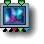25 Multi Perlin displace

parameters :
A : Amplitude (0. : 4.)
B : Octaves (1. : 128.)
C : Frequency (0. : 32.)
D : Roughness (0.12 : 0.75)

discussion :
Space transform - returns a 2D vector valued Multi perlin noise akin to
22 Multi Perlin displace
RGB texture - The returned color is procedurally generated using the displacement vector for red and green and the magnitude of displacement (length(dx,dy)) for the blue channel. The displacement amount is controlled by 'Amplitude' while 'Frequency' sets the noise base frequency.
When several layers are used with "Octaves">1 the 'Roughness' controls the fractal dimension.

##25 Disk Fractal Lab

parameters :
Algorithm slider : (0 : 25)
B : Tile # (3 : 8)
C : Parameter % (0. : 1.)

discussion :
This component provides 25 different disk fractal algorithms. Explore this component on your own. An entire chapter could be created documenting the 25 algorithms that it encapsulates. The output from a few of the algorithms is demonstrated below.

Space transform - This component can perform any of 25 different space transforms all of which have both fractal and disk-shaped aspects. The actual transform is determined by the Algorithm # parameter. Explore each of the algorithms and see how parameters B and C influence the image.

RGB texture - A color texture/pattern related to the algorithm chosen with parameter A. Many of the algorithms use hard-coded colors while others make use of the current gradient. In some cases the first auxiliary color is also used.
"Algorithm slider" parameter determines the actual algorithm used by the space transform and color texture. The parameter's values are discrete integer values and not meant to be animated continuously. "Tile #" determines the number of tiles. Each of the algorithms tiles space within the disk-shaped area in which the transform is active. Each algorithm makes use of "parameter C" in a different way. Explore the algorithms and how this parameter affects each one.

algorithms :
With contours RGB or CMY and contours Gradient the discs tends to accumulate at the circles boundaries in additive mode.
With Gradient by recursion: the circles are shaded by the level of recursion mapped into the current gradient.
• A contours RGB
• A2 RGB
• B CMY
• C1 RGB
• C2 RGB
• D RGB:
• E RGB

##25 Fractal Polar Tile

parameters :
A : Size (0. : 1.)
B : Tile # (2. : 24.)
C : Phase (-32. : 32.)

discussion :
This component transforms space with a recursive polar transform that recursively fills space with disk-shaped tiles of the incoming space. The transformed space is further divided into tiles mirrored about a rotational axis.

Space transform - See description above.

RGB texture - A pattern of disks that correspond to the tiles created by the space transform. The color is derived from the current gradient.##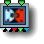25 Kleinian Group Fractal #

parameters :
A : Size (0. : 1.)
B : Tile rotation (-4. : 4.)
C : Recursions # (0 : 64)

discussion :
Kleinian Groups are a special kind of complex number fractal related to
Moebius transformations and involve matrix computation. The mathematics is quite complex, but the result is simply amazing. When the Rotation parameter is animated, the result is an interesting wobble when you are zoomed into the fractal.

Space transform - Transforms the space using one type of Kleinian group.

RGB texture - A pattern of disks that correspond to the tiles created by the space transform. The color is derived from the current gradient and first and second auxiliary colors.

Tile Rotation - When zoomed in, the system appears to wobble when rotated with Tile Rotation parameter. Recursion. Increasing the level of recursion results in the space being filled in with this fascinating fractal transform. When zoomed in, there are spiral patterns to be discovered not apparent when zoomed far out. Notice the details added when the Recursion parameter is increased.

Example: Typical Moebius spiral of packed disks using Kleinian discsExample: A 5 Branch Spirale packingalgorithms :
• Kleinian discs:
• Spirale Packing Branch 5 :
• Spirale Packing Branch 7 :
• Spirale Packing Branch 10 :

##25 Fractal Ring

parameters :
A : Radius (0.06 : 12.)
B : Tile # (2 : 16)
C : Recursions # (0 : 64)

discussion :
Fractal rings uses a set of inversion placed in a Ring like provided by the Inversion Ring tile. But Fractal Ring has its own recursion parameter and can be recursed without creating a CT.

Space transform - The interaction of the parameters can create fascinating fractal inversions that converges towards a space-filling disc packed set.

RGB texture - A pattern of disks that correspond to the tiles created by the space transform. The color is derived from the current gradient and first auxiliary color.

##25 Moebius Discs #

parameters :
A : Circle Size % (0. : 1.)
B : Rotate (0. : 90.)
C : Tiles (-16. : 16.)
D : Recursions # (1 : 256)

discussion :
Space transform - A Moebius Transformation that usually makes the set of tangent discs converge to two attractors while spiralling on two opposite sides. The parameter Recursions sets how many iteration of the functions will occur.

RGB texture - A pattern of disks that correspond to the tiles created by the space transform. The color is derived from the current gradient.

The interaction between the parameters is quite complex and should be explored while zoomed in a moderate amount. Manipulation of the K Rotate and K Tiles parameters reveals attractors and fascinating convolutions of the space as shown in the following image.algorithms :
• Single branch spiral :
Just output a single set of recursively transformed discs
• Single branch +inversions :
Creates tangent orthogonal circles that inverts the space as well.
• Double branchs+inversions :
Creates a second branch of tangent circles with inversions circles that inverts the space as well.

##25 Moebius Balls

parameters :
A : Ball Size % (0. : 1.)
B : k rotate (-3.14 : 3.14)
C : k scale (0.50 : 2.)

discussion :
This is another component based on
Moebius transformations. A series of encapsulated spaces is created that unfolds around two attractors. Complex arrangements of these Moebius Balls can be created by adjusting the K Rotate and K Scale parameters (parameters B and C).

Space transform - The incoming space is arrangement into a pattern of self-contained balls. The arrangement unfolds in a complex fashion about two attractors.

RGB texture - A pattern of disks that correspond to the space transform's tiles. The color is derived from the active gradient.

##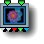25 Disk Inversion Spiral #

parameters :
A : Rotation (-3.14 : 3.14)
B : Scale (0.25 : 2.)
C : Recursion (0. : 256.)

discussion :
Space transform - A spiral arrangement of inverting circles mutually tangents that mirror the space around them. Algorithm 2 & 3 adds more branches to the main spiral.

RGB texture - A pattern of disks that correspond to the tiles created. The color is derived from the current gradient. The first auxiliary color is used to outline the disks. Rotation controls the tightness of the spiral.

Recursion is the number of disks in the spiral and the amount of transform applied.

algorithms :
• Single branch spiral :
• 4 branch spiral Alternate :
• 6 branch spiral All :

##25 Moebius Inversions

parameters :
A : Real part (-0.50 : 0.50)
B : Imaginary part (-0.50 : 0.50)
C : Recursions # (0 : 128)

discussion :
Space transform - This is another Moebius Transformation that creates stunning recursive fractal inversions of the incoming space. Adjustments to this component's three parameters can create a huge variety of patterns.

RGB texture - The dics contours are shaded in neon-green while the limit uses procedural combination of red and blue. You can implement you own shader using the transformed xy coordinates in outputs 1 and 2.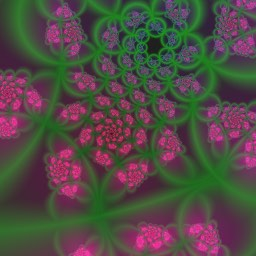##25 Random disk packing

parameters :
A : Radius (0. : 8.)
B : unused
C : Mutation (-4 : 64)

discussion :
Space transform - A recursive, fractal random tiling of space. The tiles are randomly placed and sized. Each tile is a complete recursive disk inversion. The space between tiles is filled with smaller tiles, all of which are disk inversions. The Mutation parameter lets you choose a different seed for the random values used by the packing.

RGB texture - A pattern of disks that correspond to the tiles created by the space transform. The colors are chosen randomly from the current gradient and first and second auxiliary colors.parameters :
A : Scale 0:1
B : Iterations